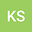loading page

Comparative Study on Fractional Isothermal Chemical Model
••Khaled M Saad
Department of Mathematics, Faculty of Applied Science, Taiz University, Department of Mathematics, College of Arts and Sciences, Kingdom of Saudi Arabia, Najran University

## Abstract

This article investigates a family of approximate solutions for the fractional isothermal chemical (FIC) equation based on mass action kinetics for autocatalytic feedback, involving the conversion of a reactant in the Liouville-Caputo sense. We apply two methods to construct numerical solutions of the FIC equation. By the first method, the spectral colloca-tion method (SCM), we reduce the FIC equation to a system of algebraic equations using Chebyshev polynomials of the third kind (CPTK). We then use the Newton-Raphson method (NRM) to solve the system of algebraic equations. By the second method, using properties of Lagrange polynomial interpolation (LPI) after applying the fundamental theorem of fractional calculus, we evaluate numerical solutions of the FIC equation. We compare these numerical solutions and compute the absolute error for varying parameter values. The results confirm the efficiency of the methods and their computationally favorable use for the numerical treatment of the model equations. 2020 Mathematics Subject Classification: 34A08; 35A22; 41A30; 65N22.